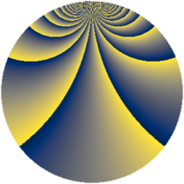# Properties

 Label 3549.2.dgLevel $3549$ Weight $2$ Character orbit 3549.dg Rep. character $\chi_{3549}(25,\cdot)$ Character field $\Q(\zeta_{78})$ Dimension $5808$ Sturm bound $970$

# Related objects

## Defining parameters

 Level: $$N$$ $$=$$ $$3549 = 3 \cdot 7 \cdot 13^{2}$$ Weight: $$k$$ $$=$$ $$2$$ Character orbit: $$[\chi]$$ $$=$$ 3549.dg (of order $$78$$ and degree $$24$$) Character conductor: $$\operatorname{cond}(\chi)$$ $$=$$ $$1183$$ Character field: $$\Q(\zeta_{78})$$ Sturm bound: $$970$$

## Dimensions

The following table gives the dimensions of various subspaces of $$M_{2}(3549, [\chi])$$.

Total New Old
Modular forms 11760 5808 5952
Cusp forms 11568 5808 5760
Eisenstein series 192 0 192

## Trace form

 $$5808q - 2q^{3} - 240q^{4} + 242q^{9} + O(q^{10})$$ $$5808q - 2q^{3} - 240q^{4} + 242q^{9} + 8q^{10} + 4q^{12} + 72q^{13} - 40q^{14} + 252q^{16} - 24q^{22} - 238q^{25} - 22q^{26} + 4q^{27} + 130q^{32} + 32q^{35} + 480q^{36} - 220q^{38} + q^{39} - 44q^{40} + 122q^{42} + 4q^{43} + 16q^{48} - 246q^{49} + 8q^{51} - 38q^{52} + 176q^{53} + 88q^{55} - 96q^{56} + 52q^{58} - 208q^{59} - 32q^{61} + 192q^{62} + 592q^{64} - 26q^{65} - 16q^{66} + 156q^{67} - 110q^{68} - 32q^{69} + 156q^{71} - 184q^{74} + 30q^{75} + 312q^{76} + 12q^{77} - 60q^{78} + 10q^{79} + 242q^{81} + 416q^{85} + 156q^{86} - 36q^{87} - 16q^{90} - 131q^{91} - 39q^{93} + 78q^{94} + 104q^{95} - 312q^{97} + O(q^{100})$$

## Decomposition of $$S_{2}^{\mathrm{new}}(3549, [\chi])$$ into newform subspaces

The newforms in this space have not yet been added to the LMFDB.

## Decomposition of $$S_{2}^{\mathrm{old}}(3549, [\chi])$$ into lower level spaces

$$S_{2}^{\mathrm{old}}(3549, [\chi]) \cong$$ $$S_{2}^{\mathrm{new}}(1183, [\chi])$$$$^{\oplus 2}$$